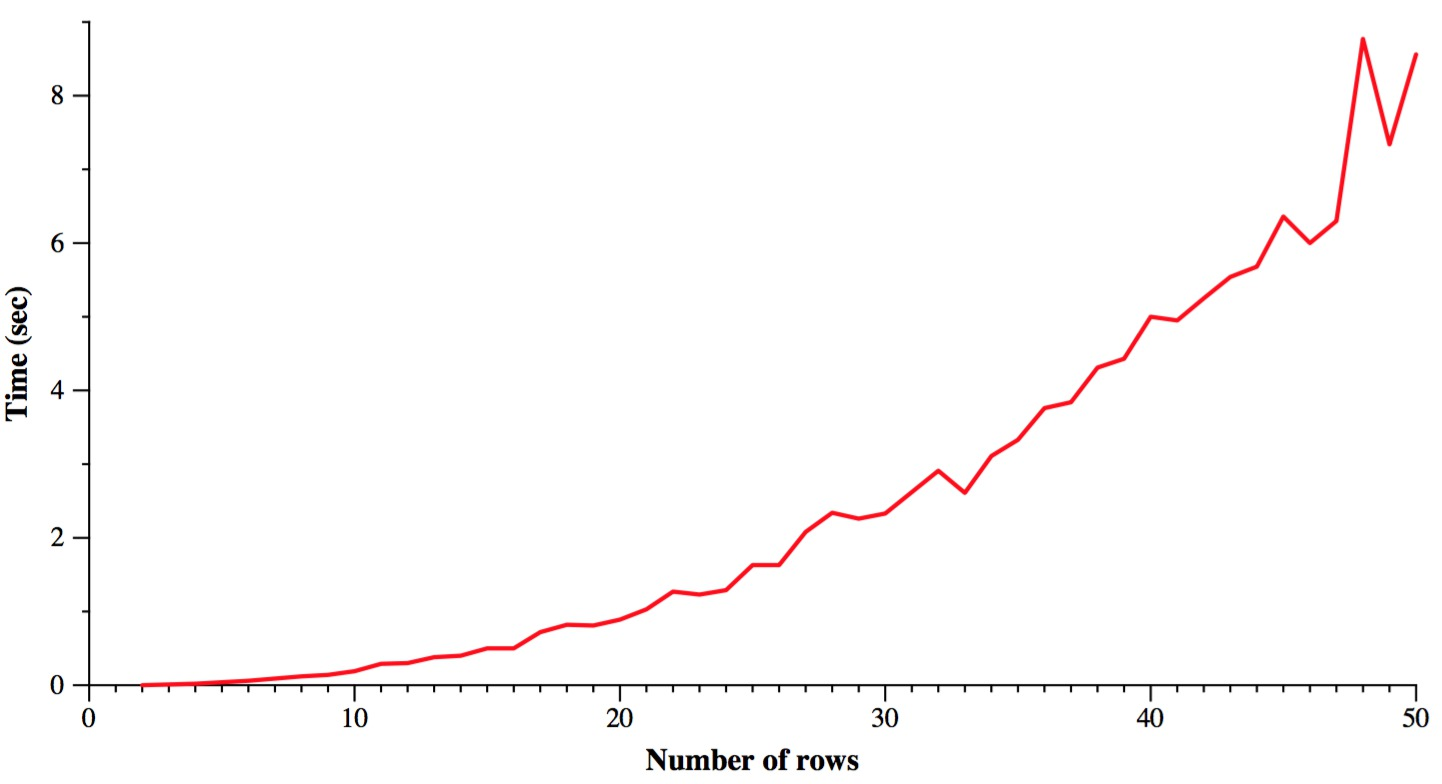## CS494 Lecture Notes - Edmonds' General Matching Algorithm (The Blossom Algorithm)

• James S. Plank
• Directory: /home/plank/cs494/Notes/Edmonds
• Original notes: December, 2017
• Most recent revision: Mon Dec 4 18:57:13 EST 2017

### Code

I have C++ code for this. Just the algorithm is in Edmonds.cpp. I have a main attached to it that does some graph generation / reading in Edmonds-Random.cpp. There's a section at the end of this writeup that talks about the code.
Wikipedia is a really good resource for this algorithm. It's how I learned it: https://en.wikipedia.org/wiki/Blossom_algorithm.

The Wikipedia page, although full of nice pictures, forces you to work through a lot of the details yourself. So, I've written these notes to help you walk through some examples. It's really the only way I could figure it out. In my description, though, I reference the line numbers of the Wikipedia algorithm. This will help you if you ever have to implement this!

### The general matching problem

You have an undirected graph. Your goal is to find a maximum matching of the graph. In other words the largest collection of edges so that no two edges share a vertex. Those of you who took CS302 from me will recall the "Word Dice" lab, where you solved the matching problem on a bipartite graph using network flow. This problem is harder, because it's on a general graph, and not a bipartite one.

### The steps of the algorithm

This is an incremental algorithm. At each step, you call FindAugmentingPath() on your graph, and a current matching. FindAugmentingPath() will return an Augmenting Path. This is a path that looks as follows (red edges are in the matching sent to FindAugmentingPath()):The two end nodes can't be part of any matching. It should be pretty clear that you can turn this path into a matching with one more edge than the previous one: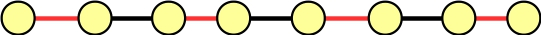The crux of the algorithm is to find such a path. You then augment the matching by one edge, and repeat. You do this until you can't find any more augmenting paths. The theoreticians have proven that this works. So, the challenging part is finding an augmenting path. The augmenting path algorithm is a pain, but I'll describe it below. You run it on a graph and a matching, and it returns a path. You can then augment the matching, and call it again on the same graph, but the new matching. You don't retain any information from one run of FindAugmentingPath() to another. They are independent. Now, in FindAugmentingPath(), You are going to partition the nodes in two ways:

• Exposed vs unexposed. Exposed means that a node is not part of the matching.
• Marked vs unmarked. Marked means that we're done processing the node. Unmarked means we haven't processed the node yet.
Edges are also labeled as marked or unmarked. When an edge is marked, you typically can consider it to be "in the forest" (see below). Or, if you want to consider it another way, a marked edge means that it will not be processed again. To start, all nodes and edges are unmarked, and nodes are determined to be exposed or unexposed.

Now, there is a notion of a Forest of trees. This forest starts out as containing one tree for each exposed node. The exposed nodes are roots of their trees. You'll note, this means that if a node is not in the forest, then it is part of the matching.

Referencing the Wikipedia algorithm, lines B02-B07 perform the initialization described in the previous two paragraphs.

You now process nodes. You are going to process nodes that have the following properties (Line B08 in the algorithm):

• They are in the forest.
• They are unmarked.
• Their distance to the root of their trees is an even number.
You'll note that at first, you'll have to process an exposed node, because those are the only nodes in the forest (and their distances from their roots are zero, which is even). If you can't find such a node, then you're done and you can't find an augmenting path.

Now, when you process a node n, you look at all of its unmarked edges (Line B09). When you're done, you'll mark the node (Line B30). For each edge, you go through the following steps:

1. If the edge is to a node m that is not in the forest, then you do the following (Lines B10-B12):

• Add the node m, and the edge from n to the forest.
• That means that n is m's parent in its tree. Since n's distance to its root is even, m's distance is odd, and m will never be processed. For that reason, you can mark it or not. In the pictures below, I mark it. Wikipedia doesn't mark it.
• Mark the edge (n,m).
• Make the realization that since m wasn't in the forest originally, it is part of a matching. Add that matching edge to the forest, and add the other node that's part of that edge's matching to the forest. Mark the edge. Don't mark the node.

2. Otherwise, the edge is to a node m that is already in the forest. If the distance of m to its root is an odd number, then you're done with this edge. Mark it and move on to the next edge (Line B14).

3. So now the edge is to a node m whose distance to its root is even. If m's root is different from n's root, then you've struck gold -- you've found an augmenting path!!!! Return it and declare success (Lines B16-B18).

4. If you're here, it means that the edge is to a node m whose root is the same as n's root, and both n and m are at even distances to the root. You have discovered an odd cycle, which goes root → n → m → root. Moreover, if this cycle has 2k+1 edges, then k of them are in the matching. This is called a blossom. What you do is contract the blossom, by deleting every node in the cycle, and replacing the cycle with a single node. Any edge from a node not in the cycle to a node in the cycle simply goes to this new node. Here's an example:Before contracting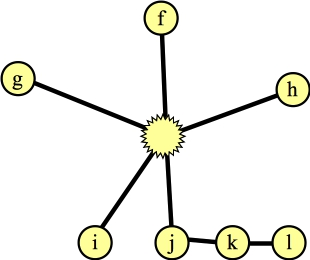After contracting

Now, find the augmenting path through this new graph (Lines B19-B24). (Whether you start over with the new graph, or continue using saved state from the old graph is something I need to think about. Wikipedia says to simply start over with the new graph. My code does that too, to be safe. However, I believe you can probably keep your old state, so long as you start processing with the blossom).

Once you find an augmenting path through this new graph, you you lift the blossom: You turn that single node back into its original nodes with their odd-size cycle. If the size of the cycle is 2k+1, then you can add k edges from the cycle to the matching. There will be exactly one way to do that.

Here's an example that you'll see later of lifting:A graph with a blossom and a matchingThe graph after lifting the blossom. There is only one way to assign those two edges in the cycle to the matching so that they work.

Of course, you may find blossoms recursively. That's not a problem -- I have an example of it later.

That's the algorithm -- wikipedia gives you an explicit algorithm, and when I refer to line numbers, they are to that algoritm.

### A non-trivial example

Here's our example graph: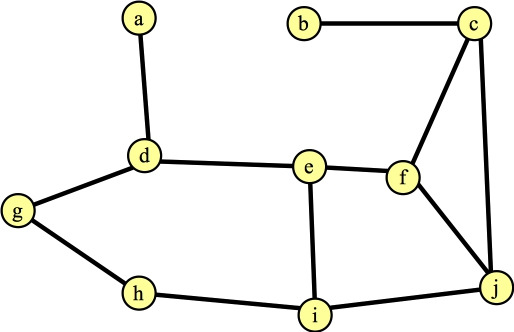Finding the first augmenting path is simple. When you go through the algorithm, you'll see that every vertex is exposed, so every vertex is the root of its own tree, and is inserted into the forest. Suppose, by happenstance, you process node d first. And suppose that the first edge you see is to e. That's a simple case (Line B16 in the Wikipedia algorithm) in the algorithm. You report the augmenting path d → e, which adds the edge (d-e) to the matching. And FindAugmentingPath() returns. Here's the graph and the matching:You'll note that if by luck, you process the edges that are in a maximum matching, then the algorithm doesn't do anything subtle. Just for yucks, let's suppose that our next calling of FindAugmentingPath() finds the path g → h, and the next one finds the path c → j. Our graph and matching now look as follows: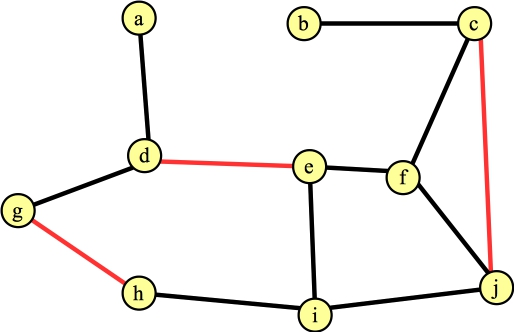We call FindAugmentingPath() again, and at this point, it helps to go through the algorithm in detail. Here's the starting state. All vertices are unmarked; all edges are also unmarked (which we'll also call "not in the forest". When we mark an edge, we are "adding it to the forest"). All exposed vertices are roots of their own trees and added to the forest. Here's the state with labeled vertices and edges: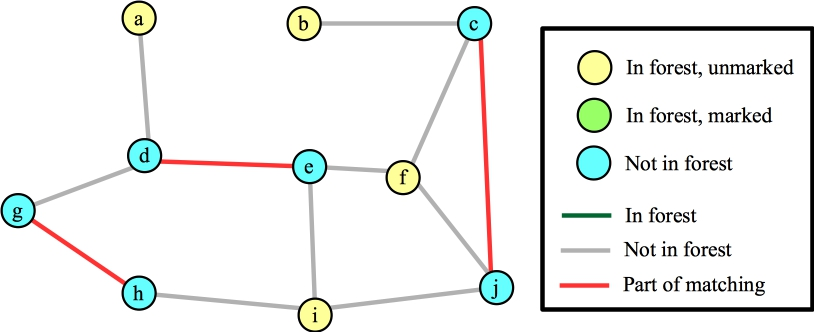Now, we'll process an exposed vertex. Let's start with b. Step B09 says to consider an unmarked edge from b. The only edge is (b,c). Since c is not in the forest (line B10), we add edges (b,c) and c's matched edge (c,j) to the tree. Vertex b has no more edges, so we mark it, and we'll move to another unmarked vertex in the forest. Here's our state. I'm deviating from the algorithm a little. If a vertex is in the forest, and its distance to the root of its tree is odd, then I'm going to mark it, because the algorithm simply ignores it (B08 specifies even).Now, suppose we process node f next. Suppose the first edge it considers is (c,f). Since c's distance to its root is odd, we do nothing (Line B14). Suppose the next edge it considers is (e,f). As above, it adds (e,f) and (d,e) to the forest. Our state is now:The last edge we consider from f is (f,j). This hits line B16: f and j are in different trees, and f's distance to its root is even (2). So, we have spotted an augmenting path: f → j → c → b. We return that path, which changes the matching to: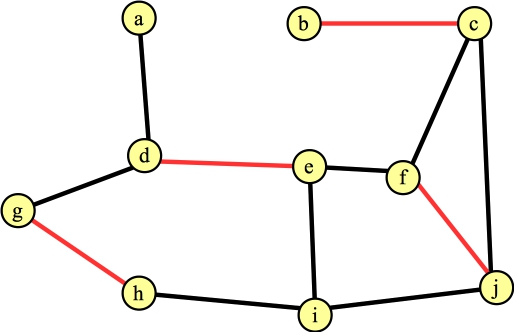You'll note, I've uncolored all of the nodes and edges (except the edges in the matching.) That's because they only apply while FindAugmentingPath() is being called.

Ok, let's call FindAugmentingPath() again. Here's its starting state: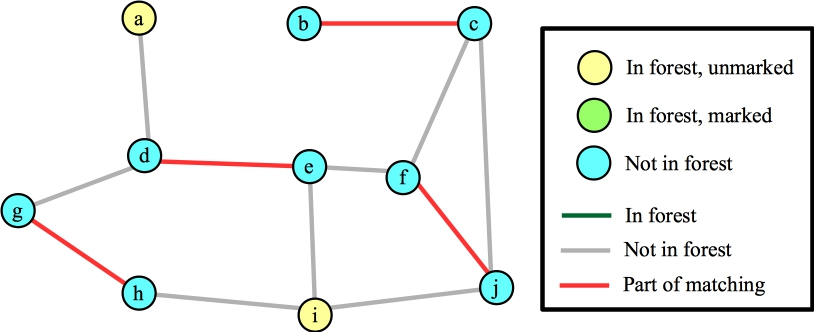Suppose we process node i first. Each of its edges are to vertices that are not in the forest, so we'll add those edges/nodes to the forest (marking them) and mark i. Here's the state when we're done with node i:Again, I've marked nodes e, h and j, because their distances to their roots is odd. Suppose the next node that I process is node g. The only edge for it to process is (d,g). This hits the else statement in line B19 of the algorithm -- nodes g and d have the same root, and their distances to that root are odd numbers. This is a blossom, so we contract the cycle into a single node. Now, the Wikipedia algorithm says to start anew by calling FindAugmentingPath() on this new graph, but you should see that we can end up at the same place, where we are processing the blossom, and we've added nodes j and f (along with edges (f,j) and (j,blossom) to the forest):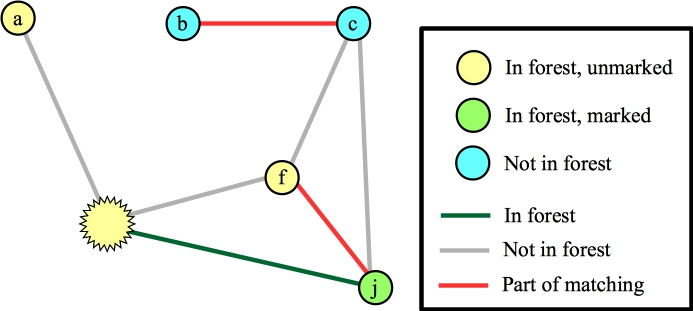Let's process the blossom, and let's look at the edge to a. We can add this edge to the matching, and what we now have is the following: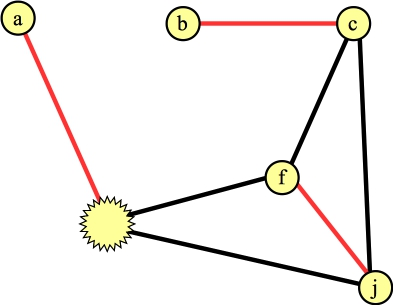We need to lift the blossom. When we do so, the vertex connected to a can't have any other edges in the matching. That determines precisely which edges inside the cycle are in the matching (it will be edges (g,h) and (e,f)):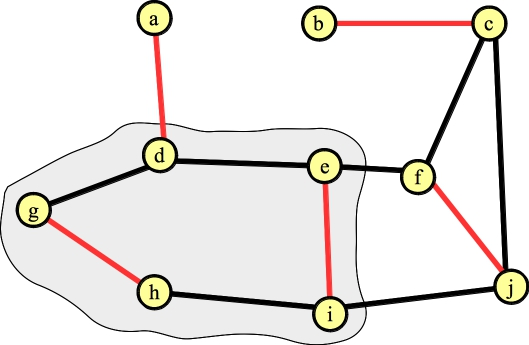We're done!

#### We could have made our lives more difficult

Let's go back to the point where we first created the blossom:Suppose that instead of processing the edge from the blossom to a we processed the edge to f. That identifies a new blossom in a three-node cycle: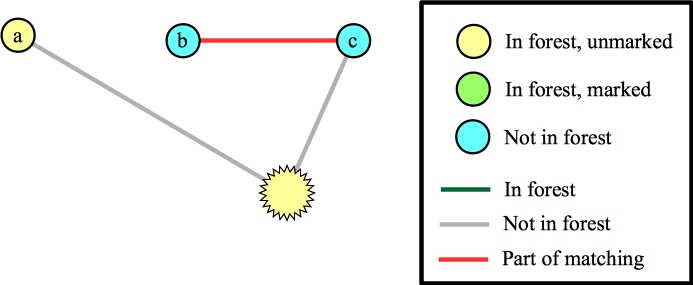Now, again we can add the edge to a to the matching: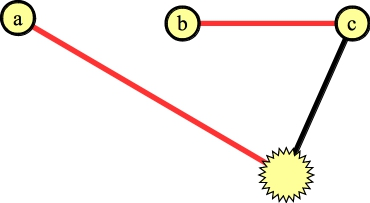Lift once -- the node in the blossom without an edge in the matching is the first blossom: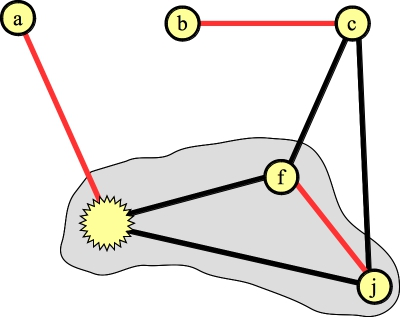And lift again to get the final matching:### What's the big deal with the blossoms?

You may find the blossoms confusing, and wonder why we need them. The reason is that they allow us to continue processing vertices without doing any backtracking -- you'll notice, there is no backtracking in this algorithm, and that's why it's fast. I'll let you think about that a little.

### Don't the blossoms mess you up in this example?

This example had me confused for a bit, so let's work through it:The point of my confusion was this: Clearly the maximum matching is not going to contain any of the edges inside that cycle. So, if you contract a blossom, doesn't that mean that you will force edges to be in the cycle? Let's get to the confusing part. Suppose our matching so far has two edges in the cycle, plus the edge (j,k). Now, we call FindAugmentingPath():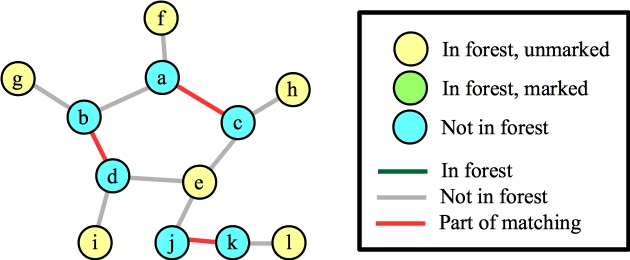Let's start with node e. This will add the three matching edges, plus the three edges to e to the forest: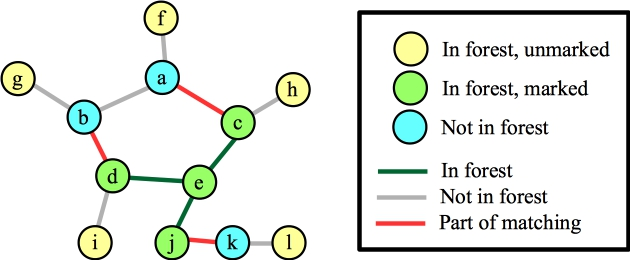Now, let's process node b, and suppose that the first edge it looks at is (a,b). It's blossom time: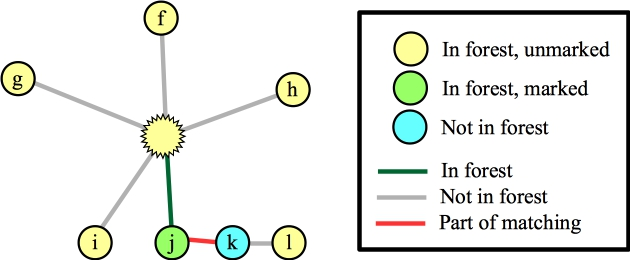Let's suppose that we process the blossom and the edge to f. That puts f into the matching, and we lift:Is that a problem? No -- we can still find augmenting paths that make this correct -- for example g → b → d → i, and then h → c → e → j → k → l. The blossom has simply gotten us to this point in the algorithm -- it has not pigeonholed us to include edges in the blossom!

### Edmonds-Random.cpp

In Edmonds.cpp, I've implemented the algorithm. You can use it pretty easily -- just call Graph::Add_Edge() to add edges to a graph, and then Graph::Find_Matching() to find the maximum matching. Read the code if you're bored -- it's not too hard, and it's not too commented... I popped a BSD license on it.

I've added a main() in In Edmonds-Random.cpp to help illustrate. Compile it and call it as follows:

```UNIX> g++ -O3 -o edmonds Edmonds-Random.cpp
UNIX> edmonds
usage: edmonds nodes edges seed(-1 to read) print(y|n|g)
UNIX>
```
The easiest way to call it is with a seed, and print equal to "y" -- it will spit out jgraph of a graph and its matching:
```UNIX> edmonds 10 15 56 y | head -n 5
newgraph
xaxis nodraw min 0 max 10 size 5
yaxis nodraw min 0 max 10 size 5
newline linethickness 2 color 0 0 0 pts 9.1571 8.7999  4.4932 9.3963
newline linethickness 2 color 1 0 0 pts 9.6312 5.3109  9.3576 2.0697
UNIX> edmonds 10 15 56 y | jgraph -P | ps2pdf - > er-example-1.pdf
UNIX>
```
Here's the graph and its matching (turning er-example-1.pdf into a JPG):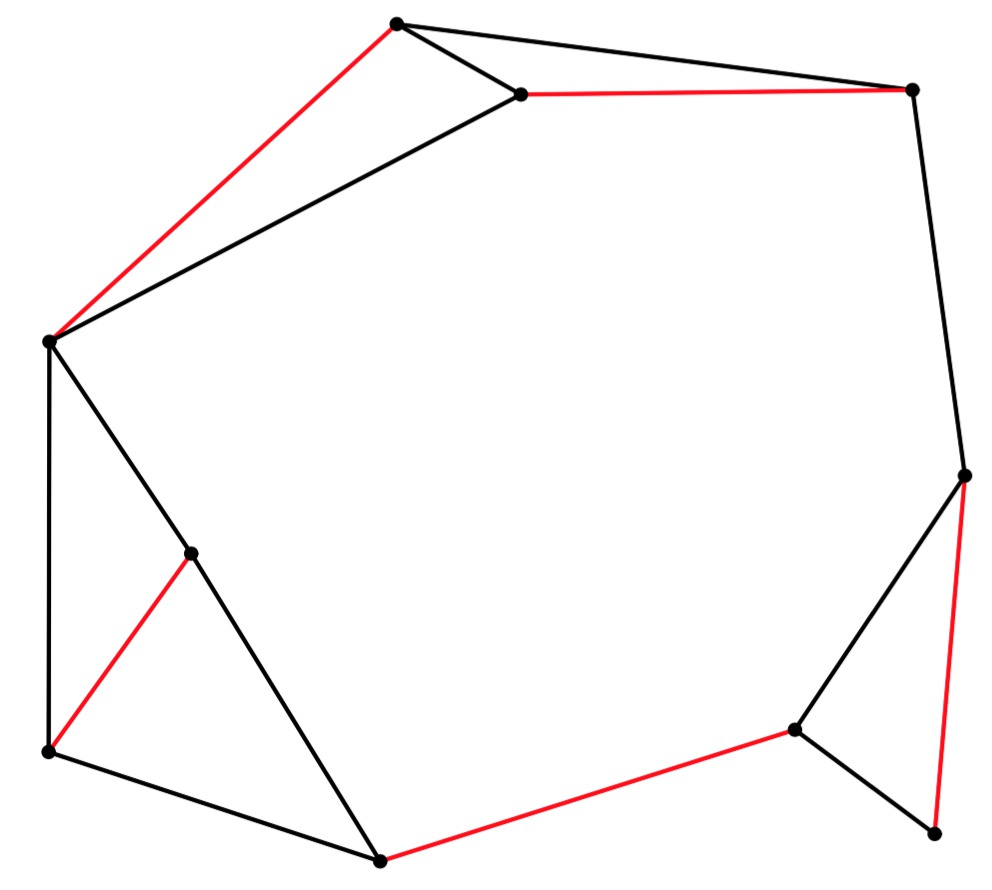If you use the "g" option, the program will print the graph in the following format:

• First the nodes, one per line, with X/Y coordinates of each node.
• A line with the word "Edges"
• The edges, one per line. Edges are integers, where the "from" node is the integer div the number of nodes, and the "to" node is the integer mod the number of nodes. For example, here's the graph above:
```UNIX> edmonds 10 15 56 g > er-example-1.txt
UNIX> cat er-example-1.txt
9.3576 2.0697
4.4932 9.3963
9.1571 8.7999
4.3433 1.8216
9.6312 5.3109
1.3518 6.5227
1.3446 2.8111
5.6165 8.7592
2.6343 4.6058
8.0947 3.0125
Edges
21
40
42
51
63
65
71
72
75
83
85
86
90
93
94
UNIX>
```
The graph from the "detailed example" above is in g1.txt. I have a slight modification in g2.txt. Here are their matchings. It is interesting to note how changing one edge completely changes the matching: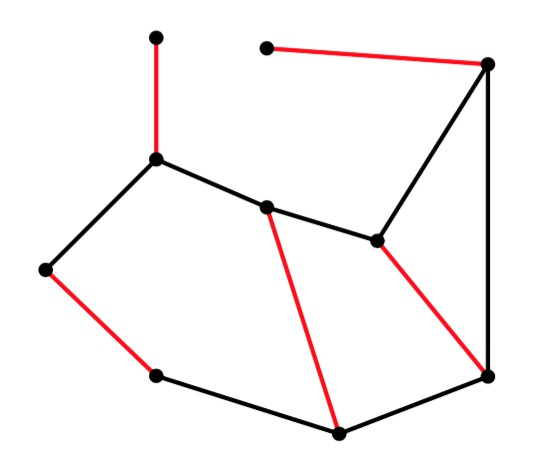g1g2

If you give the program a seed of -1, then it will read the graph from stdin. When you do that, you can give anything for the number of nodes and edges. Given that, let's have some fun. The program grid-graph.cpp generates graphs on a grid with potential edges to each node's 8 nearest neighbors. Let's see how it works on some 6x6 grids:

```UNIX> grid-graph 6 6 50 | edmonds 0 0 -1 y | jgraph -P | ps2pdf - > gg-6-6-50.pdf
UNIX> grid-graph 6 6 51 | edmonds 0 0 -1 y | jgraph -P | ps2pdf - > gg-6-6-51.pdf
UNIX> grid-graph 6 6 52 | edmonds 0 0 -1 y | jgraph -P | ps2pdf - > gg-6-6-52.pdf
```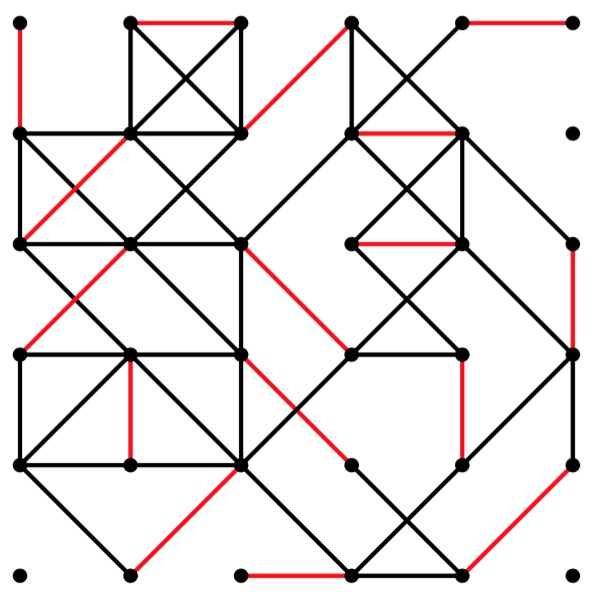gg-6-6-50.pdf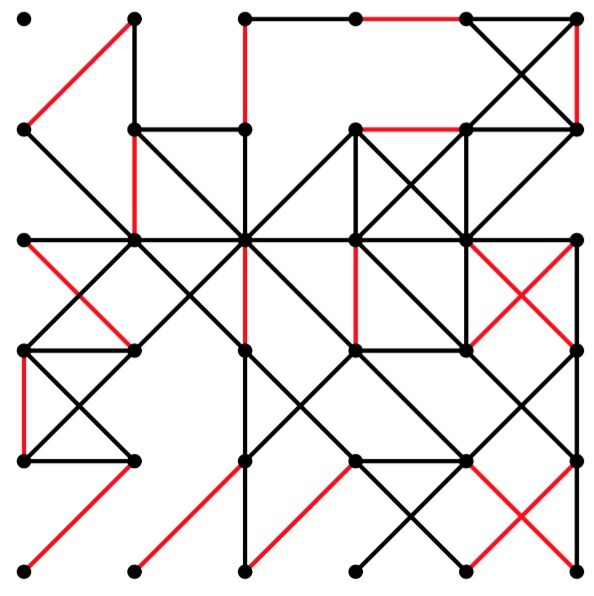gg-6-6-51.pdf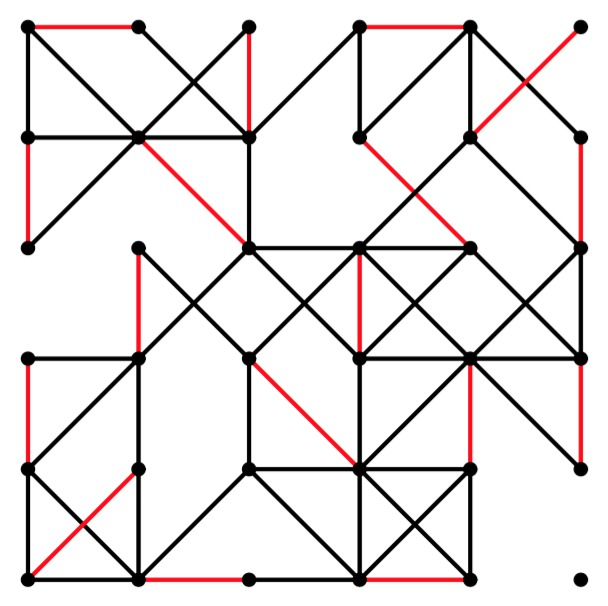gg-6-6-52.pdf

And let's try it on something big!

```UNIX> grid-graph 40 40 51 | edmonds 0 0 -1 y | sed 's/color 0 0 0/color .6 .6 .6/' | jgraph -P | ps2pdf - > gg-40-40-51.pdf
UNIX> grid-graph 80 80 66 | edmonds 0 0 -1 y | sed '/axis/s/5\$/8/' | sed 's/color 0 0 0/color .6 .6 .6/' | jgraph -P | ps2pdf - > gg-80-80-66.pdf
```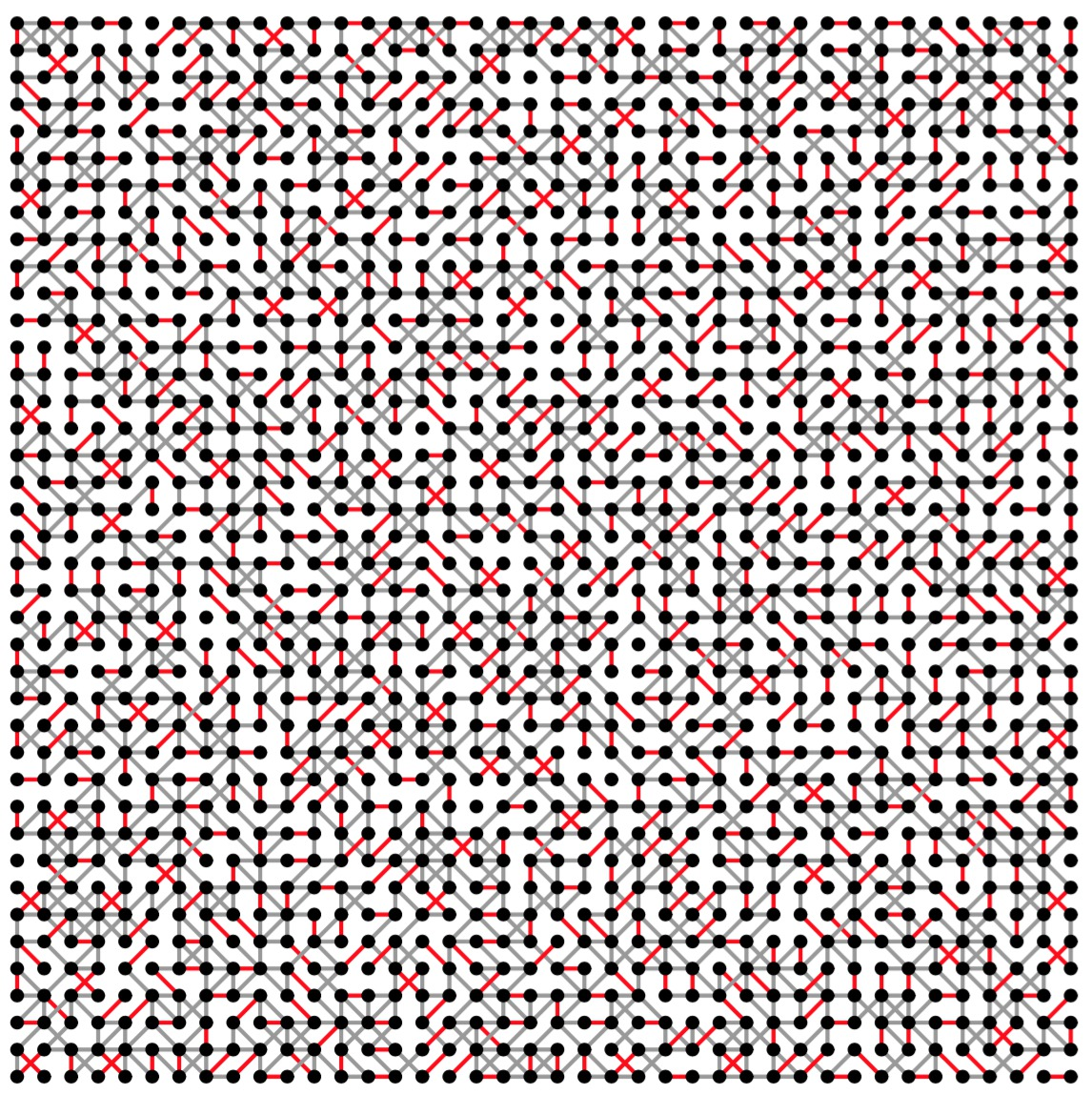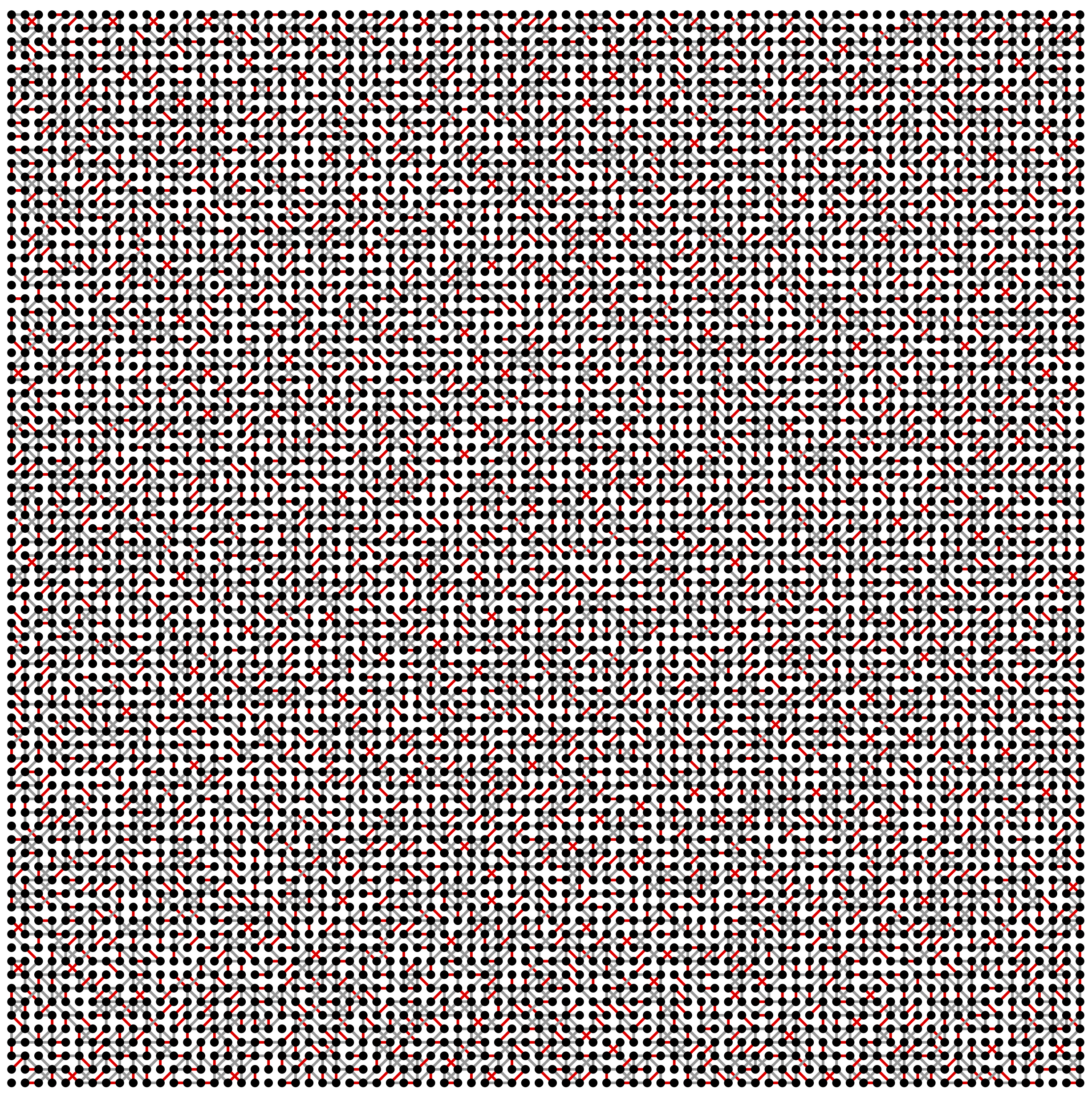Finally, let's time it. The following graph varies the number of rows from 2 to 50, while keeping the number of columns constant at 100. I only ran each test once, so you'll get some jagged lines. Plus I used a different seed for each test, so there's another source of variability. The machine is the Linux box on my desk in my office. You pretty much get what you'd expect: